# For Long Term Evolution, LTE, What is resource block in LTE? Explain the difference between refer...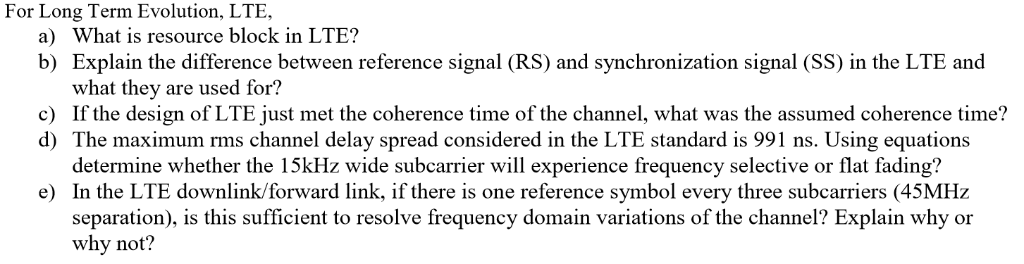For Long Term Evolution, LTE, What is resource block in LTE? Explain the difference between reference signal (RS) and synchronization signal (SS) in the LTE and what they are used for? a) b) c) If the design of LTE just met the coherence time of the channel, what was the assumed coherence time? d) The maximum rms channel delay spread considered in the LTE standard is 991 ns. Using equations determine whether the 15kHz wide subcarrier will experience frequency selective or flat fading? e) In the LTE downlink/forward link, if there is one reference symbol every three subcarriers (45MHz separation), is this sufficient to resolve frequency domain variations of the channel? Explain why or why not?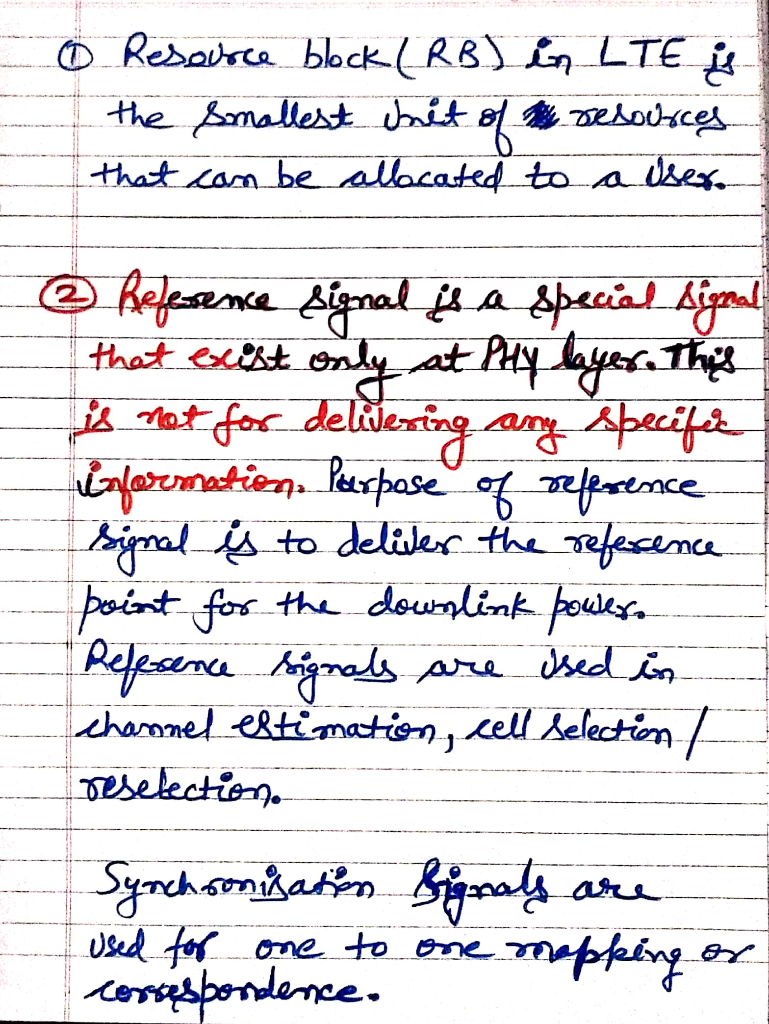##### Add Answer of: For Long Term Evolution, LTE, What is resource block in LTE? Explain the difference between refer...
Similar Homework Help Questions
• ### below is a direct sequence code division multiple access Q1 A LTE system employs orthogonal frequency division mult...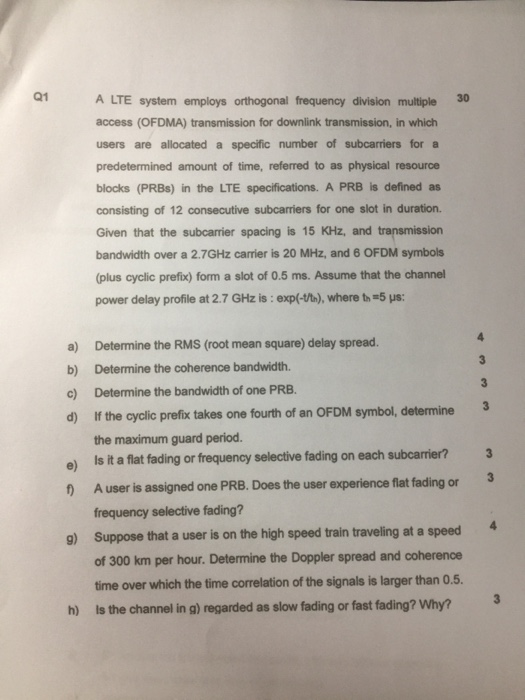below is a direct sequence code division multiple access Q1 A LTE system employs orthogonal frequency division multiple30 access (OFDMA) transmission for downlink transmission, in which users are allocated a specific number of subcarriers for a predetermined amount of time, referred to as physical resource blocks (PRBs) in the LTE specifications. A PRB is defined as consisting of 12 consecutive subcarriers for one slot in duration. Given that the subcarrier spacing is 15 KHz, and transmission bandwidth over a 2.7GHz...

• ### Determine the RMS(root mean square) delay spread. 30 Q1 A LTE system employs orthogonal frequency division multiple acc...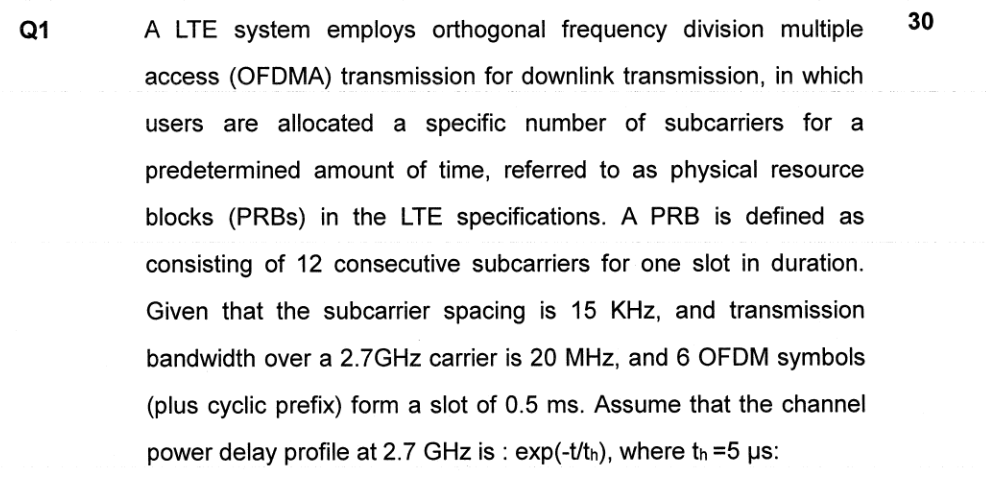Determine the RMS(root mean square) delay spread. 30 Q1 A LTE system employs orthogonal frequency division multiple access (OFDMA) transmission for downlink transmission, in which users are allocated a specific number of subcarriers for a predetermined amount of time, referred to as physical resource blocks (PRBs) in the LTE specifications. A PRB is defined as consisting of 12 consecutive subcarriers for one slot in duration. Given that the subcarrier spacing is 15 KHz, and transmission bandwidth over a 2.7GHz carrier...

• ### Problem 1: Consider the following time-invariant channel impulse response td and T are constants....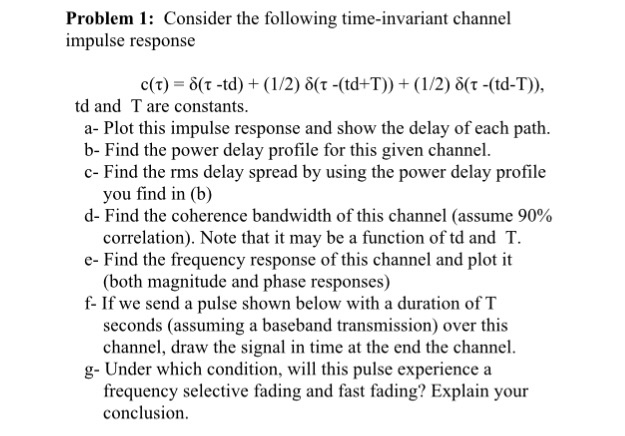Problem 1: Consider the following time-invariant channel impulse response td and T are constants. a- Plot this impulse response and show the delay of each path. b- Find the power delay profile for this given channel. c- Find the rms delay spread by using the power delay profile d-Find the coherence bandwidth of this channel (assume 90% e- Find the frequency response of this channel and plot it f- If we send a pulse shown below with a duration of...

• ### Problem 1: Consider the followingtime-invariant channel impulse response c(t)-6(t-td) + (1/2) δ(t...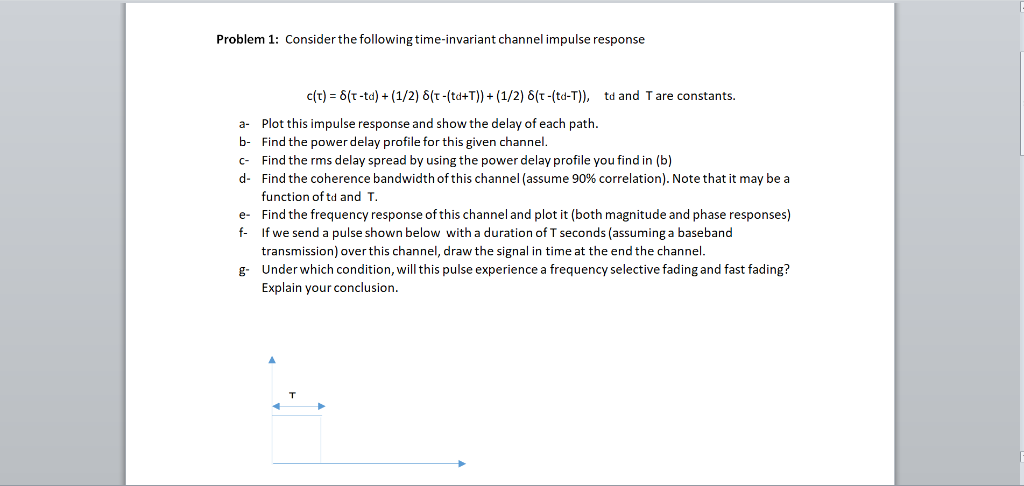Problem 1: Consider the followingtime-invariant channel impulse response c(t)-6(t-td) + (1/2) δ(t-(t0+T)) + (1/2) δ(t(td-T)), td and Tare constants. a Plot this impulse response and show the delay of each path. b- Find the power delay profile for this given channel c Find the rms delay spread by using the power delay profile you find in (b) d- Find the coherence bandwidth°fthis channel (assume 90% correlation). Note that it may be a function oftd and e Find the frequency response...

Need Online Homework Help?